﻿ 带非局部源的p-Laplace发展方程解的熄灭 Extinction for a Class of p-Laplace Evolution Equation with Nonlocal Sources

Vol.03 No.02(2014), Article ID:13605,7 pages
10.12677/AAM.2014.32018

Extinction for a Class of p-Laplace Evolution Equation with Nonlocal Sources

Haixing Zhang1*, Ruiya Hao1, Juanjuan Shen1, Shuanglong Gan1, Lang Chen2

1School of Sciences, Nantong University, Nantong

2School of Xinglin, Nantong University, Nantong

Received: Mar. 5th, 2014; revised: Apr. 1st, 2014; accepted: Apr. 8th, 2014ABSTRACT

In this article, the authors establish conditions for the extinction of nonlocal solutions, in finite time, of a class of evolution p-Laplace equation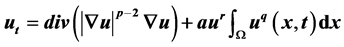with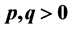, in a bounded domainwith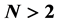.

Keywords:Fast Diffusion System, Extinction in Finite Time, Nonlinear Sources1南通大学理学院，南通

2南通大学杏林学院，南通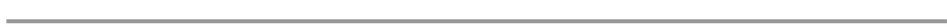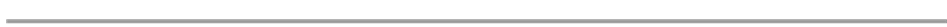1. 引言(1)(2)(3)(4)Han等在  中研究了(5)(6)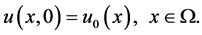(7)(8)

2. 定理1.1的证明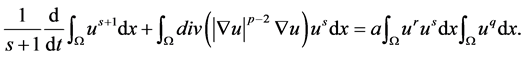(9)，则有(10)

1) 在第一种情况下，对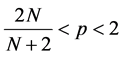在(10)中取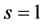，因为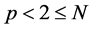，由不等式以及Sobolev嵌入定理，我们有(11)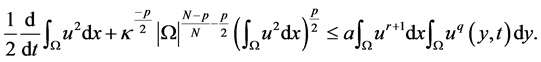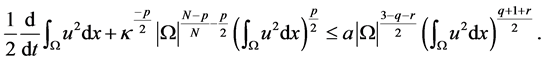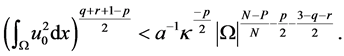(12)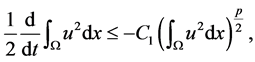(13)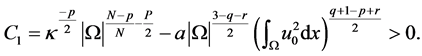(14)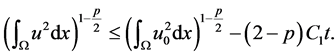（15）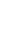(16)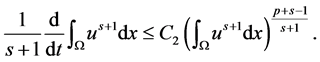(17)在有限时间(18)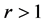或者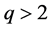时。由(1)可得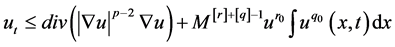(19)

Extinction for a Class of p-Laplace Evolution Equation with Nonlocal Sources. 应用数学进展,02,119-126. doi: 10.12677/AAM.2014.32018

1. 1. Kalashnikov, A.S. (1974) The propagation of disturbances in problems of nonlinear heat conduction with absorption. USSR Computational Mathematics and Mathematical Physics, 14, 70-85.

2. 2. Lair, A.V. (1993) Finite extinction time for solutions of nonlinear parabolic equations. Nonlinear Analysis: Theory, Methods & Applications, 21, 1-8.

3. 3. Lair, A.V. and Oxley, M.E. (1994) Extinction in finite time for a nonlinear absorption diffusion equation. Journal of Mathematical Analysis and Applications, 182, 857-866.

4. 4. Lair, A.V. and Oxley, M.E. (1994) Finite extinction time for a nonlinear parabolic Neumann boundary value problem. Applied Analysis, 55, 41-50.

5. 5. 顾永耕 (1994) 抛物型方程的解熄灭的充要条件. 数学学报, 37, 73-79.

6. 6. Li, Y.X. and Wu, J.C. (2005) Extinction for fast diffusion equations with nonlinear sources. Electronic Journal of Dif- ferential Equations, 2005, 1-7.

7. 7. Wang, M.X. and Wang, Y.M. (1996) Properties of positive solutions for non-local reaction-diffusion problems. Ma- thematical Methods in the Applied Sciences, 19, 1141-1156.

8. 8. Lin, Z.G. and Liu, Y.R. (2004) Uniform blow up profiles for diffusion equations with nonlocal source and nonlocal boundary. Acta Mathematica Scientia, 24, 443-450.

9. 9. 陈玉娟 (2006) 非局部的退化抛物型方程组的解的爆破和整体存在性. 数学物理学报, 26A, 731-740.

10. 10. Fang, Z.B. and Xu, X.H. (2012) Extinction behavior of solutions for the p-Laplacian equations with nonlocal sources. Nonlinear Analysis: Real World Applications, 13, 1780-1789.

11. 11. Han, Y.Z. and Gao, W.J. (2011) Extinction for a fast diffusion equation with a nonlinear nonlocal source. Archiv der Mathematik, 97, 353-363.

12. 12. Tian, Y. and Mu, C.L. (2008) Extinction and non-extinction for a p-Laplacian equation with nonlinear source. Nonlinear Analysis, 69, 2422-2431.